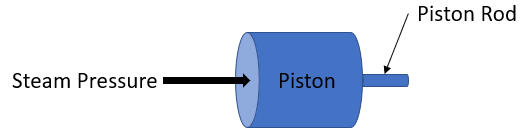Consider a steam engine's piston has a 10 in diameter, and a 1 in diameter piston rod. If the piston's rod has a design stress of 1,000 psi, what is the max steam gauge pressure in psi?Expand Hint
$$Pressure =\frac{Force}{Area}$$$Hint 2 Since the piston force is the same force on the piston's rod, uniaxial loading is applied: $$\sigma =\frac{F}{A}$$$
where $$\sigma$$ is the stress on the cross section, $$F$$ is the loading, and $$A$$ is the cross sectional area.
$$\sigma =\frac{F}{A}$$$where $$\sigma$$ is the stress on the cross section, $$F$$ is the loading, and $$A$$ is the cross sectional area. $$F_{rod}=\sigma A_{rod}=(1,000\frac{lb}{in^2})(\frac{\pi}{4})(1in)^2=785\:lb$$$
$$\sum F=(pressure)(area)=(P)(\frac{\pi}{4} \cdot D^2)$$$$$F_{piston}=(P_{gauge})[\frac{\pi}{4} \cdot (10in)^2]$$$
Because $$F_{piston}=F_{rod}$$ :
$$785lb=(P_{gauge})[\frac{\pi}{4} \cdot 100in^2]$$$Finding the max steam gauge pressure: $$P_{gauge}=\frac{785lb}{\frac{\pi}{4} \cdot 100in^2}=10\:psi$$$